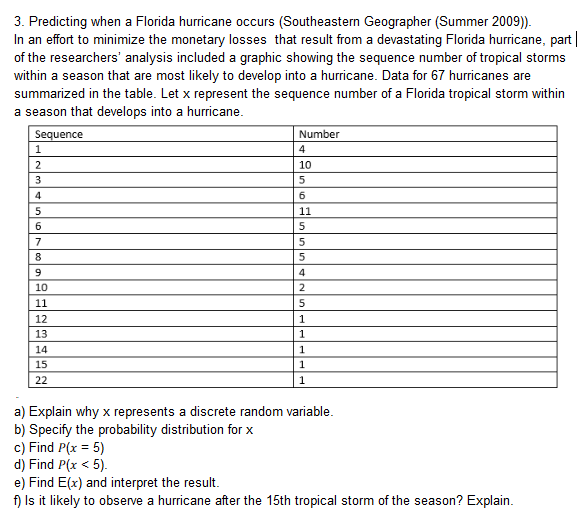3. Predicting when a Florida hurricane occurs (Southeastern Geographer (Summer 2009))In an effort to minimize the monetary losses that result from a devastating Florida hurricane, partof the researchers' analysis included a graphic showing the sequence number of tropical stormswithin a season that are most likely to develop into a hurricane. Data for 67 hurricanes aresummarized in the table. Let x represent the sequence number of a Florida tropical storm withina season that develops into a hurricaneenceNumber1012131415a) Explain why x represents a discrete random variableb) Specify the probability distribution for xc) Find P(x-5)d) Find P(x < 5)e) Find E(x) and interpret the resultf) Is it likely to observe a hurricane after the 15th tropical storm of the season? Explain

Questionhelp_outlineImage Transcriptionclose3. Predicting when a Florida hurricane occurs (Southeastern Geographer (Summer 2009)) In an effort to minimize the monetary losses that result from a devastating Florida hurricane, part of the researchers' analysis included a graphic showing the sequence number of tropical storms within a season that are most likely to develop into a hurricane. Data for 67 hurricanes are summarized in the table. Let x represent the sequence number of a Florida tropical storm within a season that develops into a hurricane ence Number 10 12 13 14 15 a) Explain why x represents a discrete random variable b) Specify the probability distribution for x c) Find P(x-5) d) Find P(x < 5) e) Find E(x) and interpret the result f) Is it likely to observe a hurricane after the 15th tropical storm of the season? Explain fullscreen
Step 1

a.

Explain the reason behind representing x as discrete random variable:

Discrete random variable:

If the variable X takes finite or countable values, then the variable X is said to be discrete.

The data represents the values of number of hurricanes developed corresponding to the sequence number of tropical storms within a season.

Consider the variable x represents the sequence number of tropical storms within a season.

The variable sequence number of tropical storms within a season takes the countable or finite values. The sequence numbers of tropical storms within a season are 1, 2, 3, 4, 5, 6, 7, 8, 9, 10, 11, 12, 13, 14, 15 and 22.

Hence, the variable sequence number of tropical storms within a season is said to be discrete.

Step 2

Construct the probability distribution for the variable x.

Conditions of the discrete probability distribution:

• The probabilities of all the values of the variable X must lie between 0 and 1.
• The sum of probabilities of all the values of X must be equal to “1”.

The total number of developed hurricanes is n = 67.

The general formula to obtain the probability of an event is,

Probability of an event = (Number of favourable cases for the event)/(Total number of cases).

Here, the probabilities for the values of the variable x is obtained as,

Probability of xi = (Number of hurricanes developed for that particular xi)/(Total number of hurricanes).

The probabilities for the values x = 1, 2, 3, 4, 5, 6 is obtained below:

Step 3

Construct the probability distribution for the variable x.

The probabilities for the v...

Want to see the full answer?

See Solution

Want to see this answer and more?

Our solutions are written by experts, many with advanced degrees, and available 24/7

See Solution
Tagged in

Other